Scalar product - Defination

Chapter 10 Class 12 Vector Algebra
Concept wise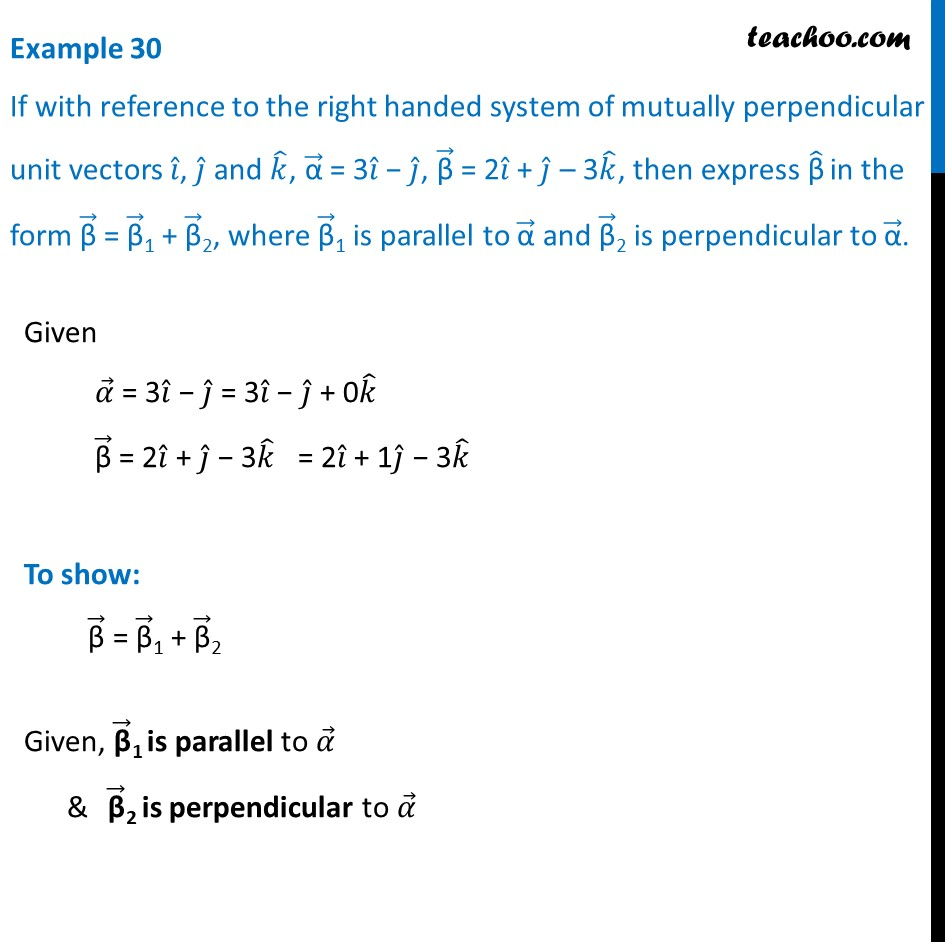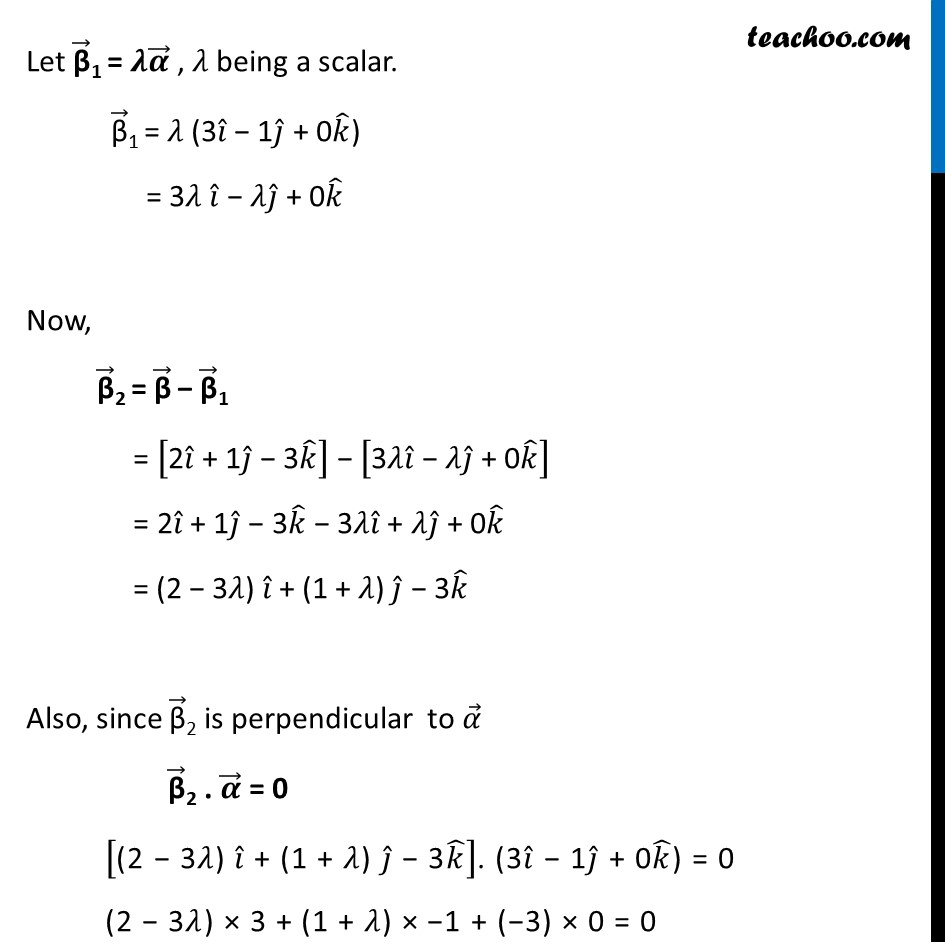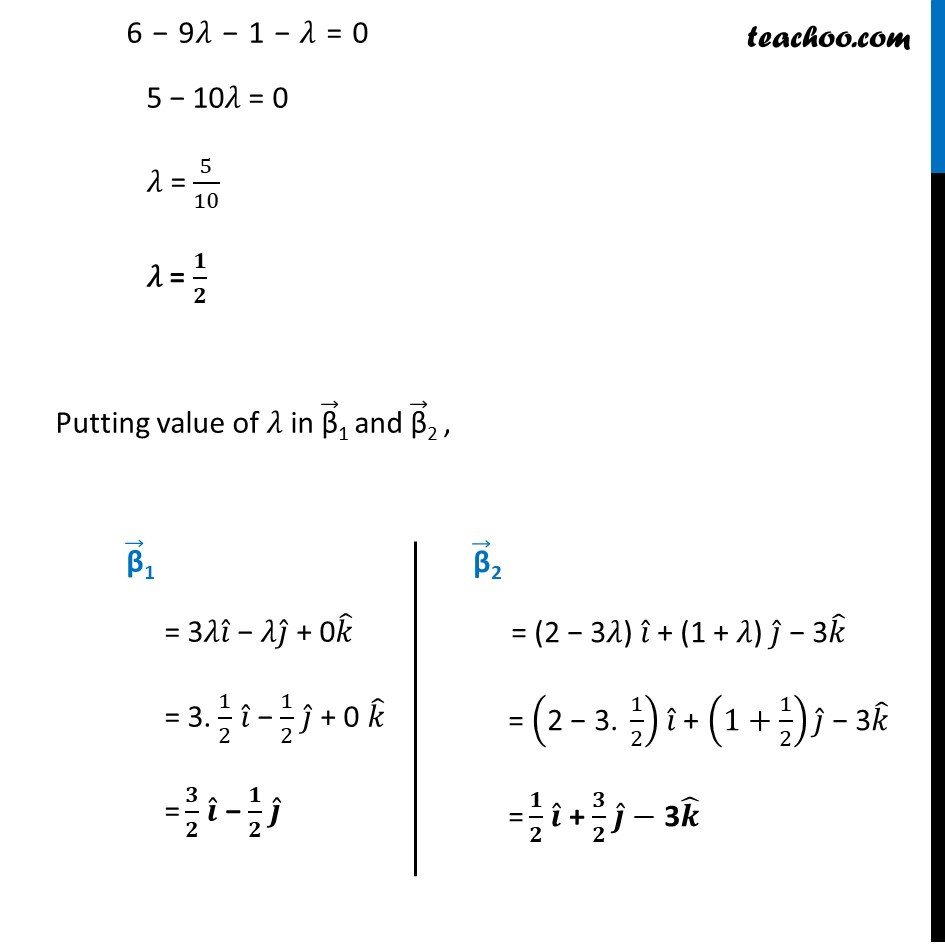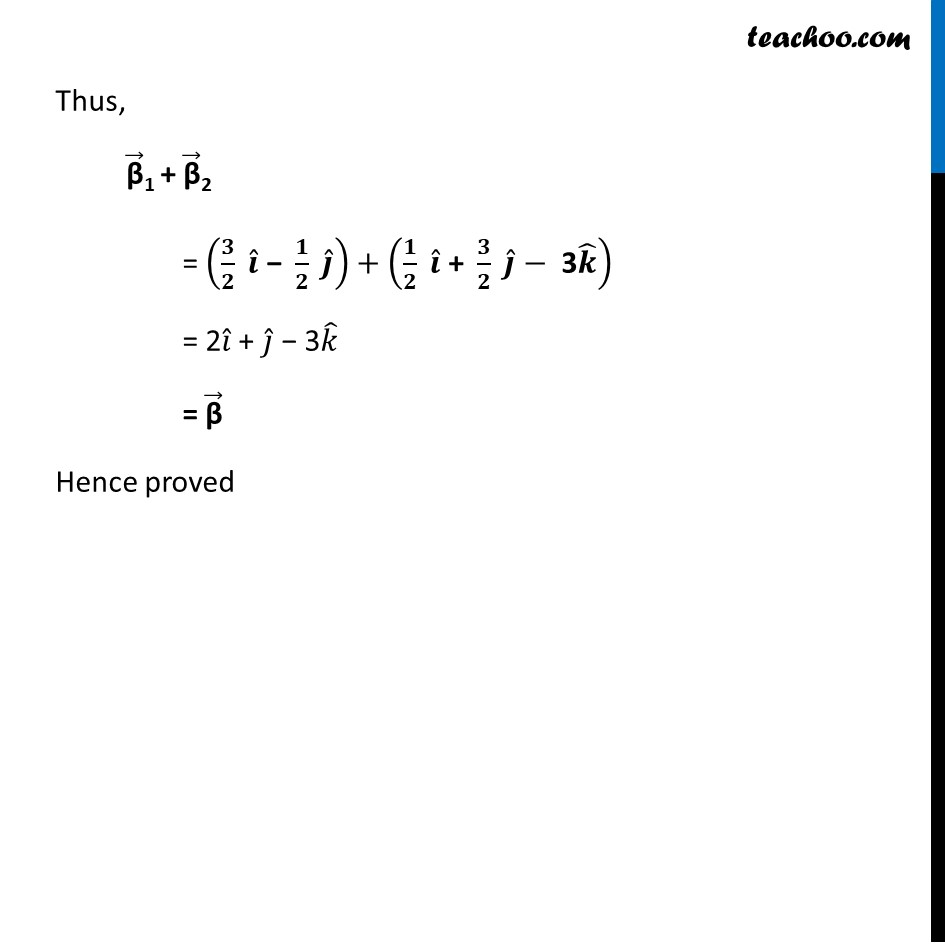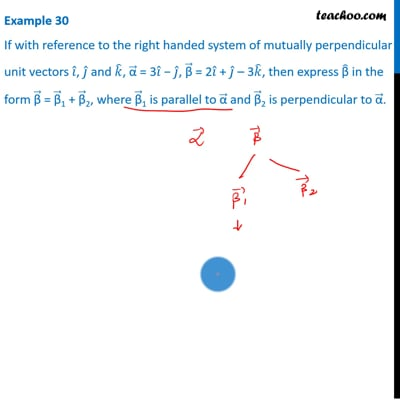This video is only available for Teachoo black users

Introducing your new favourite teacher - Teachoo Black, at only ₹83 per month

### Transcript

Example 30 If with reference to the right handed system of mutually perpendicular unit vectors 𝑖 ̂, 𝑗 ̂ and 𝑘 ̂, "α" ⃗ = 3𝑖 ̂ − 𝑗 ̂, "β" ⃗ = 2𝑖 ̂ + 𝑗 ̂ – 3𝑘 ̂, then express "β" ̂ in the form "β" ⃗ = "β" ⃗1 + "β" ⃗2, where "β" ⃗1 is parallel to "α" ⃗ and "β" ⃗2 is perpendicular to "α" ⃗.Given 𝛼 ⃗ = 3𝑖 ̂ − 𝑗 ̂ = 3𝑖 ̂ − 𝑗 ̂ + 0𝑘 ̂ "β" ⃗ = 2𝑖 ̂ + 𝑗 ̂ − 3𝑘 ̂ = 2𝑖 ̂ + 1𝑗 ̂ − 3𝑘 ̂ To show: "β" ⃗ = "β" ⃗1 + "β" ⃗2 Given, "β" ⃗1 is parallel to 𝛼 ⃗ & "β" ⃗2 is perpendicular to 𝛼 ⃗ Let "β" ⃗1 = 𝝀𝜶 ⃗ , 𝜆 being a scalar. "β" ⃗1 = 𝜆 (3𝑖 ̂ − 1𝑗 ̂ + 0𝑘 ̂) = 3𝜆 𝑖 ̂ − 𝜆𝑗 ̂ + 0𝑘 ̂ Now, "β" ⃗2 = "β" ⃗ − "β" ⃗1 = ["2" 𝑖 ̂" + 1" 𝑗 ̂" − 3" 𝑘 ̂ ] − ["3" 𝜆𝑖 ̂" − 𝜆" 𝑗 ̂" + 0" 𝑘 ̂ ] = 2𝑖 ̂ + 1𝑗 ̂ − 3𝑘 ̂ − 3𝜆𝑖 ̂ + 𝜆𝑗 ̂ + 0𝑘 ̂ = (2 − 3𝜆) 𝑖 ̂ + (1 + 𝜆) 𝑗 ̂ − 3𝑘 ̂ Also, since "β" ⃗2 is perpendicular to 𝛼 ⃗ "β" ⃗2 . 𝜶 ⃗ = 0 ["(2 − 3𝜆) " 𝑖 ̂" + (1 + 𝜆) " 𝑗 ̂" − 3" 𝑘 ̂ ]. (3𝑖 ̂ − 1𝑗 ̂ + 0𝑘 ̂) = 0 (2 − "3𝜆") × 3 + (1 + 𝜆) × −1 + (−3) × 0 = 0 6 − 9"𝜆" − 1 − 𝜆 = 0 5 − 10𝜆 = 0 𝜆 = 5/10 𝜆 = 𝟏/𝟐 Putting value of 𝜆 in "β" ⃗1 and "β" ⃗2 , "β" ⃗1 = 3𝜆𝑖 ̂ − 𝜆𝑗 ̂ + 0𝑘 ̂ = 3. 1/2 𝑖 ̂ − 1/2 𝑗 ̂ + 0 𝑘 ̂ = 𝟑/𝟐 𝒊 ̂ − 𝟏/𝟐 𝒋 ̂ "β" ⃗2 = (2 − 3𝜆) 𝑖 ̂ + (1 + 𝜆) 𝑗 ̂ − 3𝑘 ̂ = ("2 − 3. " 1/2) 𝑖 ̂ + (1+1/2) 𝑗 ̂ − 3𝑘 ̂ = 𝟏/𝟐 𝒊 ̂ + 𝟑/𝟐 𝒋 ̂− 3𝒌 ̂ "β" ⃗2 = (2 − 3𝜆) 𝑖 ̂ + (1 + 𝜆) 𝑗 ̂ − 3𝑘 ̂ = ("2 − 3. " 1/2) 𝑖 ̂ + (1+1/2) 𝑗 ̂ − 3𝑘 ̂ = 𝟏/𝟐 𝒊 ̂ + 𝟑/𝟐 𝒋 ̂− 3𝒌 ̂ Thus, "β" ⃗1 + "β" ⃗2 = (𝟑/𝟐 " " 𝒊 ̂" − " 𝟏/𝟐 " " 𝒋 ̂ )+(𝟏/𝟐 " " 𝒊 ̂" + " 𝟑/𝟐 " " 𝒋 ̂−" 3" 𝒌 ̂ ) = 2𝑖 ̂ + 𝑗 ̂ − 3𝑘 ̂ = "β" ⃗ Hence proved